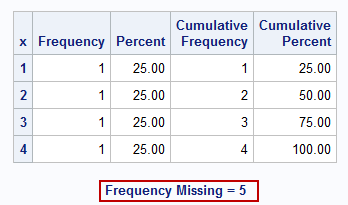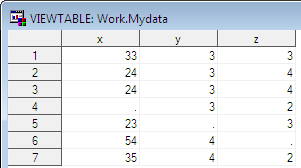# Missing Values in SAS

In SAS, Numeric and Character missing values are represented differently.

## Numeric Missing Values

SAS stores 28 types of missing values in a numeric variable. They are as follows :

1. dot-underscore . _
2. dot .
3. .A through .Z ( Not case sensitive)

Sorting Order : dot- underscore is the lowest valued missing value. After the dot-underscore, comes the dot, and then the dot-A. The dot-Z is the highest valued missing value.

Run the following code and see how SAS treats them missing value
```data temp;
input x;
cards;
1
2
3
.
.A
.X
.Z
._
4
;
run;

proc freq;
table x;
run;
```## SAS: Check for missing numeric values

The following code checks for dot missing value only. It does not check for other 27 special numeric missing values (._ , .A through .Z)

```data outdata;
set temp;
length y \$12;
If x=. then y = "Missing";
else y = "Non-Missing";
run;
```

The following code checks for all 28 numeric missing values (. , ._ , .A through .Z)

```data outdata;
set temp;
length y \$12;
If x<=.z then y = "Missing";
else y = "Non-Missing";
run;
```

The MISSING function accepts both character and numeric variables and returns the value 1 if the variable contains a missing value or zero otherwise.

```data outdata;
set temp;
length y \$12;
If missing(x) then y = "Missing";
else y = "Non-Missing";
run;
```

## SAS: Check for missing character values

Character missing values are represented by a single blank enclosed in quotes ' '.

```DATA mydata;
INPUT Product \$;
DATALINES;
ProductA
.
ProductA
ProductA
.
ProductB
ProductB
;
RUN;

data outdata;
set mydata;
length y \$12;
If missing(Product) then y = "Missing";
else y = "Non-Missing";
run;
```

## SUM Function vs. + Operator for Missing Values

Suppose we have a data set containing three variables - X, Y and Z. They all have some missing values. We wish to compute sum of all the variables.```data mydata;
input x y z;
datalines;
1 . 3
4 5 .
. 8 9
;
run;

data mydata2;
set mydata;
a=sum(x,y,z);
p=x+y+z;
run;
```

The SUM function returns the sum of non-missing values whereas "+" operator returns a missing value if any of the values are missing.## Functions that handle MISSING data

NMISS : The NMISS() function will return the number of missing values in the specified list of numeric variables. The NMISS() function will convert any character values to numeric before assessing if the argument value is missing.

CMISS : The CMISS() function counts the number of missing values for both character and numeric variables without requiring character values to be converted to numeric.

N : The N() function returns the number of non-missing values in a list of numeric variables.

```/* Create a sample SAS dataset */
data sample_dataset;
input var1 var2 var3 \$;
datalines;
10  20  A
15   .  B
.  25  C
12  18   .
.   .  D
;

/* Calculate the number of missing values for each variable */
data missing_values;
set sample_dataset;

/* Use NMISS function to count missing numeric values */
num_missing_var1 = NMISS(var1);
num_missing_var1_var2 = NMISS(var1, var2);

/* Use CMISS function to count missing values */
missing_all = CMISS(var1, var2, var3);

/* Use N function to count total non-missing values for numeric values*/
num_non_missing = N(var1, var2, var3);
total_non_missing = 3-missing_all;

run;

/* Print the resulting dataset */
proc print data=missing_values noobs;
run;
```

## How to Count Missing Values in a Dataset

To count missing values in a SAS dataset, you can use the NMISS option in PROC MEANS procedures in SAS.

```/* Create a sample SAS dataset with missing values */
DATA mydata;
INPUT ID Age Income;
DATALINES;
1 25 50000
2 . 60000
3 30 .
4 40 75000
5 22 40000
. 28 55000
7 35 65000
8 . .
9 19 30000
;
RUN;

PROC MEANS DATA=mydata NMISS;
RUN;
```
CALL MISSING

SAS provides the statement CALL MISSING() to explicitly initialise or set a variable value to be missing.

```data _null_;
call missing( num_1, num_2, x );
num_3 = x;
put num_1 = / num_2 = / num_3 = ;
run;
```

See result in the Log Window. In the above SAS code, a data step is used to initialize and assign missing values to three variables (num_1, num_2, and x) using the call missing statement. Then, the value of x is assigned to num_3, and the values of num_1, num_2, and num_3 are printed using the put statement. Since num_1 and num_2 have been explicitly set to missing, their output will show as missing, while num_3 will take the value of x, which is also missing.

Delete empty rows
options missing = ' ';
set outdata;
if missing(cats(of _all_)) then delete;
run;

## How missing values are handled in SAS procedures

1. PROC FREQ

To count missing values for categorical variables, you can use the PROC FREQ procedure. By default, PROC FREQ excludes missing values and percentages are based on the number of non-missing values. If you use the "/ MISSING" option in the tables statement, the percentages are based on the total number of observations (non-missing and missing) and the percentage of missing values are reported in the table.

```DATA mydata;
INPUT Product \$;
DATALINES;
ProductA
.
ProductA
ProductA
.
ProductB
ProductB
;
RUN;

PROC FREQ DATA=mydata;
TABLES Product / MISSING;
RUN;
```
2. PROC MEANS

It produces statistics on non-missing data only. The NMISS option is used to calculate number of missing values.
Proc Means Data = test N NMISS;
Var q1 - q5 ;
Run;
To see number of observations having a missing value for the classification variable, type MISSING option in PROC MEANS.
Proc Means data = test N NMISS MISSING;
Class Age ;
Var q1 - q5;
Run;
3. PROC CORR

By default, correlations are computed based on the number of pairs with non-missing data (pairwise deletion of missing data). The nomiss option can be used on the proc corr statement to request that correlations be computed only for observations that have non-missing data for all variables on the var statement (listwise deletion of missing data).

4. PROC REG

If any of the variables on the model or var statement are missing, they are excluded from the analysis (i.e., listwise deletion of missing data)

5. PROC LOGISTIC

If any of the variables on the model or var statement are missing, they are excluded from the analysis (i.e., listwise deletion of missing data)

6. PROC FACTOR

Missing values are deleted listwise, i.e., observations with missing values on any of the variables in the analysis are omitted from the analysis.
Related Posts
ShareDeepanshu founded ListenData with a simple objective - Make analytics easy to understand and follow. He has over 10 years of experience in data science. During his tenure, he worked with global clients in various domains like Banking, Insurance, Private Equity, Telecom and HR.

12 Responses to "Missing Values in SAS"
1.Thanks a lot! This blog has been very helpful.

2.Thanks, the information was really helpful.

3.I tried this code with the temp data but its not removing any rows.

options missing = ' ';
set outdata;
if missing(cats(of _all_)) then delete;
run;

Output is same as the data set. Is it working for any one else ?

1.i tried the same code

data work.missvalue;
input x y z;
cards;
1 2 4
7 8 .
7 - 7
6 9 .
9 8 _
9 9 7
;
run;

options missing='';
set work.missvalue;
IF MISSING(CATS(OF_ALL_))THEN DELETE;
RUN;

but in my above code out is blank : Rows 4 and Column 0

4.Please edit this chapter based on the fact that some of the datasets are dependent on other chapters and we can't run the code to see what's going on (unless we still have prior datasets)

1.BrO,Why dont you help in doing the same.Rather then pointing someone,do some good for others as well.

Mr.Deepanshu is giving his best for all beginners.

5.Hi....,
I would like to get the variables has to be printed which have couple of missing values.

For ex: if the name variable has missing record the name variable should be printed.

if id variables has a missing record the id variables has to be printed.

6.thanks for the excellent tutorials , please keep datasets and examples in same lesson. it will be helpful for indevedual topic readers.

7.data nidhi;
input x y z;
cards;
22 5 56
. 25 96
56 . 56
66 52 41
96 52 .
85 . 52
. . 44
;
run;
I want to assign 0 to the all missing values in one statment.
can anyone help?

8.Try this out ....
data nidhi1;set nidhi;
array new _numeric_;
do over new;
if new=. then new=0;
end;
run;

9.I want to treat 43% missing with value=1,27% missing with value=2, 16% missing with value=3, 14% missing with value=4.
How can I do this?
10.